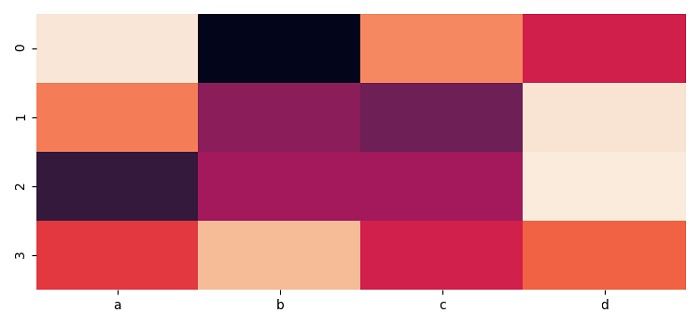# How to hide the colorbar of a Seaborn heatmap?

To hide the colorbar of a Seaborn heatmap, we can use cbar=False in heatmap() method.

## Steps

• Set the figure size and adjust the padding between and around the subplots.

• Make a dataframe using 4 columns.

• Use heatmap() method to plot rectangular data as a color-encoded matrix.

• To display the figure, use show() method.

## Example

import seaborn as sns
import pandas as pd
import numpy as np
import matplotlib.pyplot as plt

plt.rcParams["figure.figsize"] = [7.50, 3.50]
plt.rcParams["figure.autolayout"] = True

df = pd.DataFrame(np.random.random((4, 4)), columns=["a", "b", "c", "d"])
sns.heatmap(df, cbar=False)

plt.show()

## Output